+关注继续查看

A.1 ndarray对象的内部机理

NumPy的ndarray提供了一种将同质数据块（可以是连续或跨越）解释为多维数组对象的方式。正如你之前所看到的那样，数据类型（dtype）决定了数据的解释方式，比如浮点数、整数、布尔值等。

ndarray如此强大的部分原因是所有数组对象都是数据块的一个跨度视图（strided view）。你可能想知道数组视图arr[::2,::-1]不复制任何数据的原因是什么。简单地说，ndarray不只是一块内存和一个dtype，它还有跨度信息，这使得数组能以各种步幅（step size）在内存中移动。更准确地讲，ndarray内部由以下内容组成：

• 一个指向数据（内存或内存映射文件中的一块数据）的指针。
• 数据类型或dtype，描述在数组中的固定大小值的格子。
• 一个表示数组形状（shape）的元组。
• 一个跨度元组（stride），其中的整数指的是为了前进到当前维度下一个元素需要“跨过”的字节数。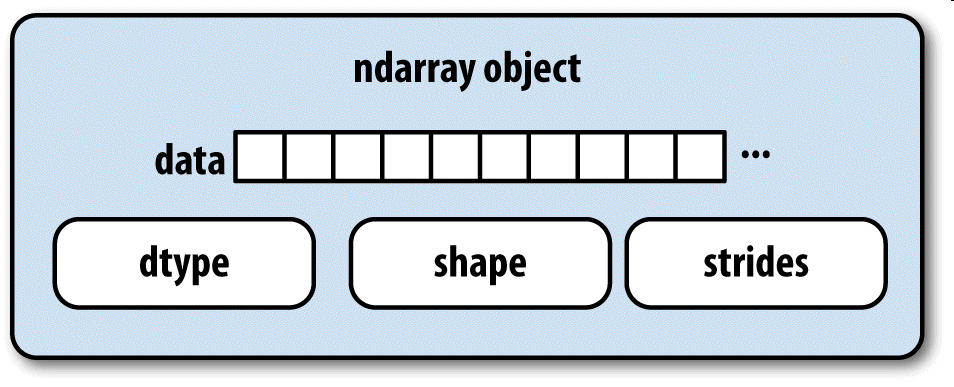In : np.ones((10, 5)).shape
Out: (10, 5)

In : np.ones((3, 4, 5), dtype=np.float64).strides
Out: (160, 40, 8)

NumPy数据类型体系

In : ints = np.ones(10, dtype=np.uint16)

In : floats = np.ones(10, dtype=np.float32)

In : np.issubdtype(ints.dtype, np.integer)
Out: True

In : np.issubdtype(floats.dtype, np.floating)
Out: True

In : np.float64.mro()
Out:
[numpy.float64,
numpy.floating,
numpy.inexact,
numpy.number,
numpy.generic,
float,
object]

In : np.issubdtype(ints.dtype, np.number)
Out: True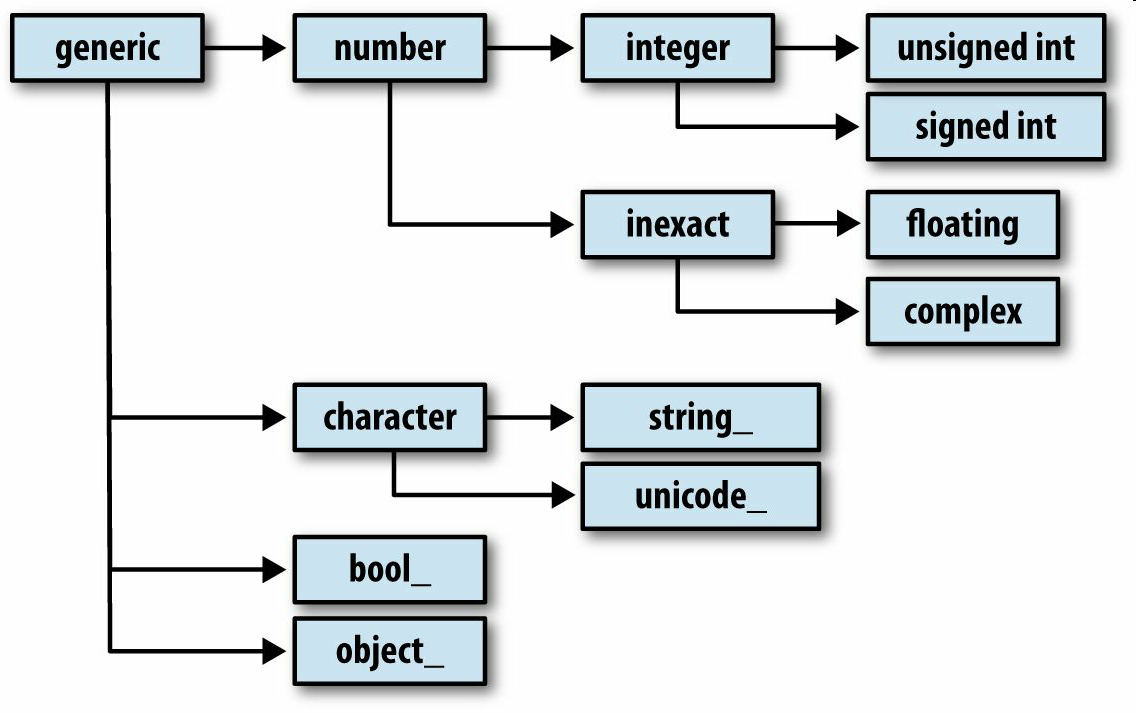A.2 高级数组操作

数组重塑

In : arr = np.arange(8)

In : arr
Out: array([0, 1, 2, 3, 4, 5, 6, 7])

In : arr.reshape((4, 2))
Out:
array([[0, 1],
[2, 3],
[4, 5],
[6, 7]])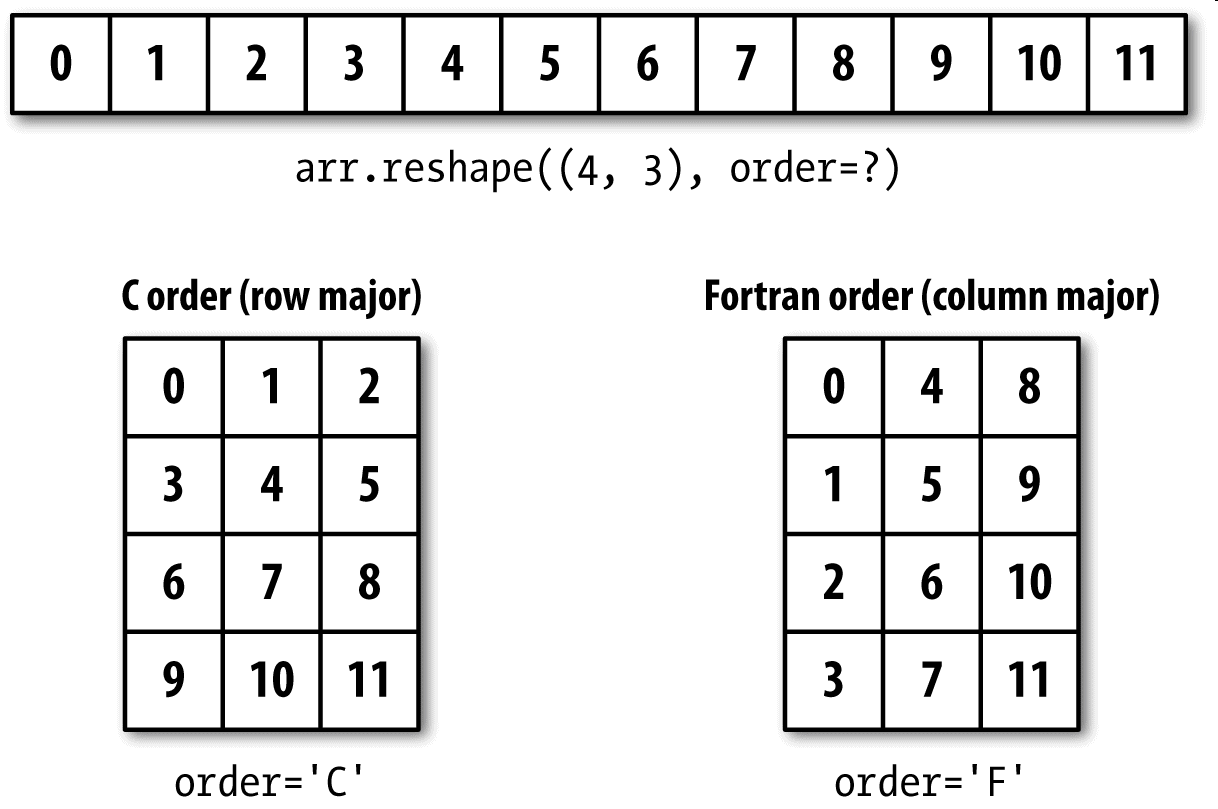In : arr.reshape((4, 2)).reshape((2, 4))
Out:
array([[0, 1, 2, 3],
[4, 5, 6, 7]])

In : arr = np.arange(15)

In : arr.reshape((5, -1))
Out:
array([[ 0,  1,  2],
[ 3,  4,  5],
[ 6,  7,  8],
[ 9, 10, 11],
[12, 13, 14]])

In : arr = np.arange(15).reshape((5, 3))

In : arr
Out:
array([[ 0,  1,  2],
[ 3,  4,  5],
[ 6,  7,  8],
[ 9, 10, 11],
[12, 13, 14]])

In : arr.ravel()
Out: array([ 0,  1,  2,  3,  4,  5,  6,  7,  8,  9, 10, 11, 12, 13, 14])

In : arr.flatten()
Out: array([ 0,  1,  2,  3,  4,  5,  6,  7,  8,  9, 10, 11, 12, 13, 14])

C和Fortran顺序

NumPy允许你更为灵活地控制数据在内存中的布局。默认情况下，NumPy数组是按行优先顺序创建的。在空间方面，这就意味着，对于一个二维数组，每行中的数据项是被存放在相邻内存位置上的。另一种顺序是列优先顺序，它意味着每列中的数据项是被存放在相邻内存位置上的。

In : arr = np.arange(12).reshape((3, 4))

In : arr
Out:
array([[ 0,  1,  2,  3],
[ 4,  5,  6,  7],
[ 8,  9, 10, 11]])

In : arr.ravel()
Out: array([ 0,  1,  2,  3,  4,  5,  6,  7,  8,  9, 10, 11])

In : arr.ravel('F')
Out: array([ 0,  4,  8,  1,  5,  9,  2,  6, 10,  3,  7, 11])• C/行优先顺序：先经过更高的维度（例如，轴1会先于轴0被处理）。
• Fortran/列优先顺序：后经过更高的维度（例如，轴0会先于轴1被处理）。

数组的合并和拆分

numpy.concatenate可以按指定轴将一个由数组组成的序列（如元组、列表等）连接到一起：

In : arr1 = np.array([[1, 2, 3], [4, 5, 6]])

In : arr2 = np.array([[7, 8, 9], [10, 11, 12]])

In : np.concatenate([arr1, arr2], axis=0)
Out:
array([[ 1,  2,  3],
[ 4,  5,  6],
[ 7,  8,  9],
[10, 11, 12]])

In : np.concatenate([arr1, arr2], axis=1)
Out:
array([[ 1,  2,  3,  7,  8,  9],
[ 4,  5,  6, 10, 11, 12]])

In : np.vstack((arr1, arr2))
Out:
array([[ 1,  2,  3],
[ 4,  5,  6],
[ 7,  8,  9],
[10, 11, 12]])

In : np.hstack((arr1, arr2))
Out:
array([[ 1,  2,  3,  7,  8,  9],
[ 4,  5,  6, 10, 11, 12]])

In : arr = np.random.randn(5, 2)

In : arr
Out:
array([[-0.2047,  0.4789],
[-0.5194, -0.5557],
[ 1.9658,  1.3934],
[ 0.0929,  0.2817],
[ 0.769 ,  1.2464]])

In : first, second, third = np.split(arr, [1, 3])

In : first
Out: array([[-0.2047,  0.4789]])

In : second
Out:
array([[-0.5194, -0.5557],
[ 1.9658,  1.3934]])
In : third
Out:
array([[ 0.0929,  0.2817],
[ 0.769 ,  1.2464]])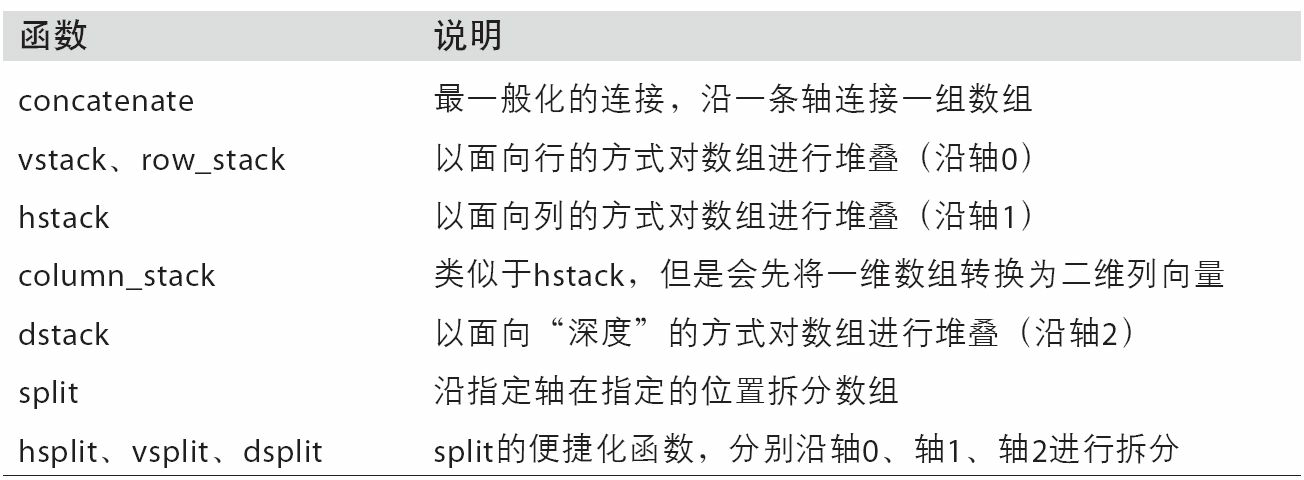堆叠辅助类：r_和c_

NumPy命名空间中有两个特殊的对象——r_和c_，它们可以使数组的堆叠操作更为简洁：

In : arr = np.arange(6)

In : arr1 = arr.reshape((3, 2))

In : arr2 = np.random.randn(3, 2)

In : np.r_[arr1, arr2]
Out:
array([[ 0.    ,  1.    ],
[ 2.    ,  3.    ],
[ 4.    ,  5.    ],
[ 1.0072, -1.2962],
[ 0.275 ,  0.2289],
[ 1.3529,  0.8864]])

In : np.c_[np.r_[arr1, arr2], arr]
Out:
array([[ 0.    ,  1.    ,  0.    ],
[ 2.    ,  3.    ,  1.    ],
[ 4.    ,  5.    ,  2.    ],
[ 1.0072, -1.2962,  3.    ],
[ 0.275 ,  0.2289,  4.    ],
[ 1.3529,  0.8864,  5.    ]])

In : np.c_[1:6, -10:-5]
Out:
array([[  1, -10],
[  2,  -9],
[  3,  -8],
[  4,  -7],
[  5,  -6]])

r_和c_的具体功能请参考其文档。

元素的重复操作：tile和repeat

In : arr = np.arange(3)

In : arr
Out: array([0, 1, 2])

In : arr.repeat(3)
Out: array([0, 0, 0, 1, 1, 1, 2, 2, 2])

In : arr.repeat([2, 3, 4])
Out: array([0, 0, 1, 1, 1, 2, 2, 2, 2])

In : arr = np.random.randn(2, 2)

In : arr
Out:
array([[-2.0016, -0.3718],
[ 1.669 , -0.4386]])

In : arr.repeat(2, axis=0)
Out:
array([[-2.0016, -0.3718],
[-2.0016, -0.3718],
[ 1.669 , -0.4386],
[ 1.669 , -0.4386]])

In : arr.repeat([2, 3], axis=0)
Out:
array([[-2.0016, -0.3718],
[-2.0016, -0.3718],
[ 1.669 , -0.4386],
[ 1.669 , -0.4386],
[ 1.669 , -0.4386]])

In : arr.repeat([2, 3], axis=1)
Out:
array([[-2.0016, -2.0016, -0.3718, -0.3718, -0.3718],
[ 1.669 ,  1.669 , -0.4386, -0.4386, -0.4386]])

tile的功能是沿指定轴向堆叠数组的副本。你可以形象地将其想象成“铺瓷砖”：

In : arr
Out:
array([[-2.0016, -0.3718],
[ 1.669 , -0.4386]])

In : np.tile(arr, 2)
Out:
array([[-2.0016, -0.3718, -2.0016, -0.3718],
[ 1.669 , -0.4386,  1.669 , -0.4386]])

In : arr
Out:
array([[-2.0016, -0.3718],
[ 1.669 , -0.4386]])

In : np.tile(arr, (2, 1))
Out:
array([[-2.0016, -0.3718],
[ 1.669 , -0.4386],
[-2.0016, -0.3718],
[ 1.669 , -0.4386]])

In : np.tile(arr, (3, 2))
Out:
array([[-2.0016, -0.3718, -2.0016, -0.3718],
[ 1.669 , -0.4386,  1.669 , -0.4386],
[-2.0016, -0.3718, -2.0016, -0.3718],
[ 1.669 , -0.4386,  1.669 , -0.4386],
[-2.0016, -0.3718, -2.0016, -0.3718],
[ 1.669 , -0.4386,  1.669 , -0.4386]])

花式索引的等价函数：take和put

In : arr = np.arange(10) * 100

In : inds = [7, 1, 2, 6]

In : arr[inds]
Out: array([700, 100, 200, 600])

ndarray还有其它方法用于获取单个轴向上的选区：

In : arr.take(inds)
Out: array([700, 100, 200, 600])

In : arr.put(inds, 42)

In : arr
Out: array([  0,  42,  42, 300, 400, 500,  42,  42,800, 900])

In : arr.put(inds, [40, 41, 42, 43])

In : arr
Out: array([  0,  41,  42, 300, 400, 500,  43,  40, 800, 900])

In : inds = [2, 0, 2, 1]

In : arr = np.random.randn(2, 4)

In : arr
Out:
array([[-0.5397,  0.477 ,  3.2489, -1.0212],
[-0.5771,  0.1241,  0.3026,  0.5238]])

In : arr.take(inds, axis=1)
Out:
array([[ 3.2489, -0.5397,  3.2489,  0.477 ],
[ 0.3026, -0.5771,  0.3026,  0.1241]])

put不接受axis参数，它只会在数组的扁平化版本（一维，C顺序）上进行索引。因此，在需要用其他轴向的索引设置元素时，最好还是使用花式索引。

A.3 广播

In : arr = np.arange(5)

In : arr
Out: array([0, 1, 2, 3, 4])

In : arr * 4
Out: array([ 0,  4,  8, 12, 16])

In : arr = np.random.randn(4, 3)

In : arr.mean(0)
Out: array([-0.3928, -0.3824, -0.8768])

In : demeaned = arr - arr.mean(0)

In : demeaned
Out:
array([[ 0.3937,  1.7263,  0.1633],
[-0.4384, -1.9878, -0.9839],
[-0.468 ,  0.9426, -0.3891],
[ 0.5126, -0.6811,  1.2097]])

In : demeaned.mean(0)
Out: array([-0.,  0., -0.])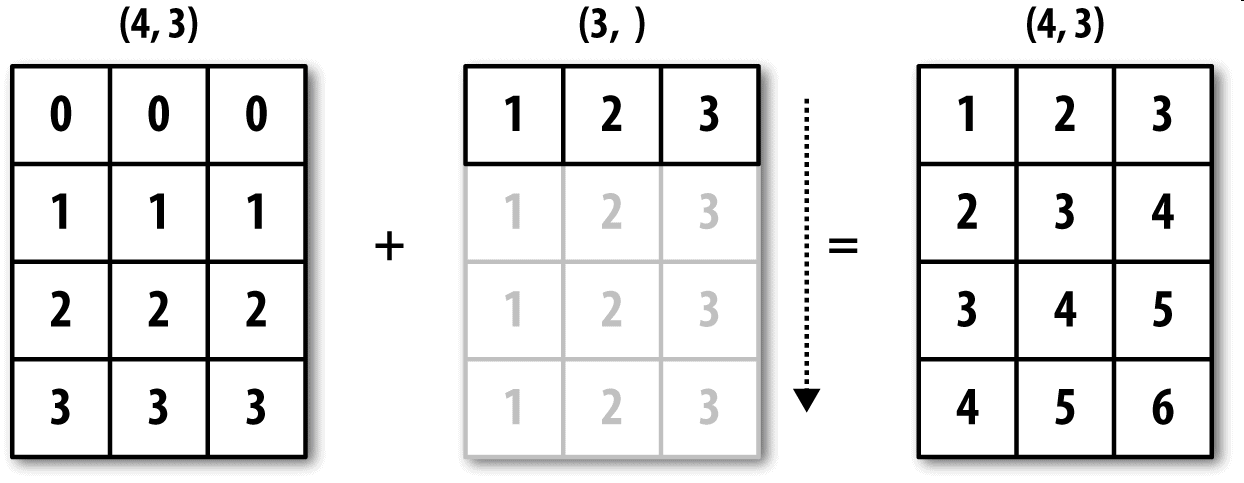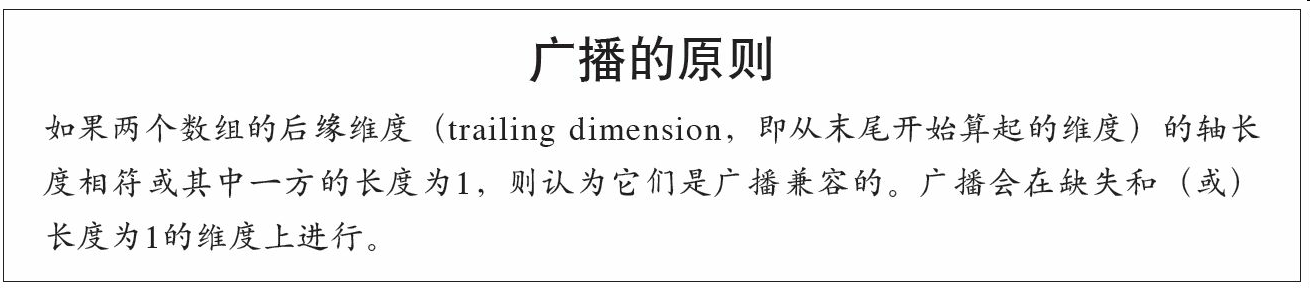In : arr
Out:
array([[ 0.0009,  1.3438, -0.7135],
[-0.8312, -2.3702, -1.8608],
[-0.8608,  0.5601, -1.2659],
[ 0.1198, -1.0635,  0.3329]])

In : row_means = arr.mean(1)

In : row_means.shape
Out: (4,)

In : row_means.reshape((4, 1))
Out:
array([[ 0.2104],
[-1.6874],
[-0.5222],
[-0.2036]])

In : demeaned = arr - row_means.reshape((4, 1))

In : demeaned.mean(1)
Out: array([ 0., -0.,  0.,  0.])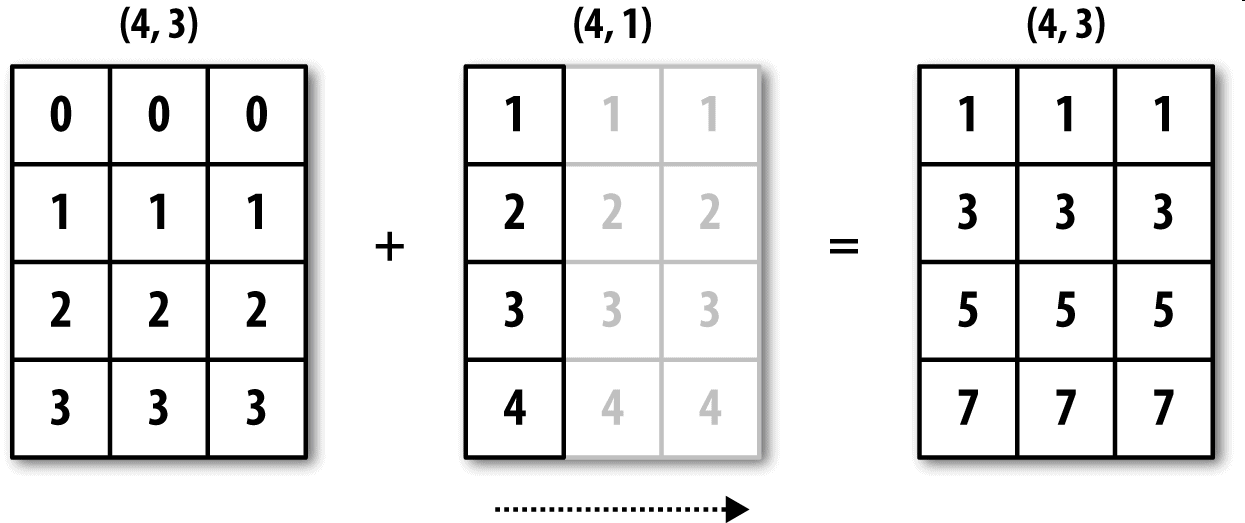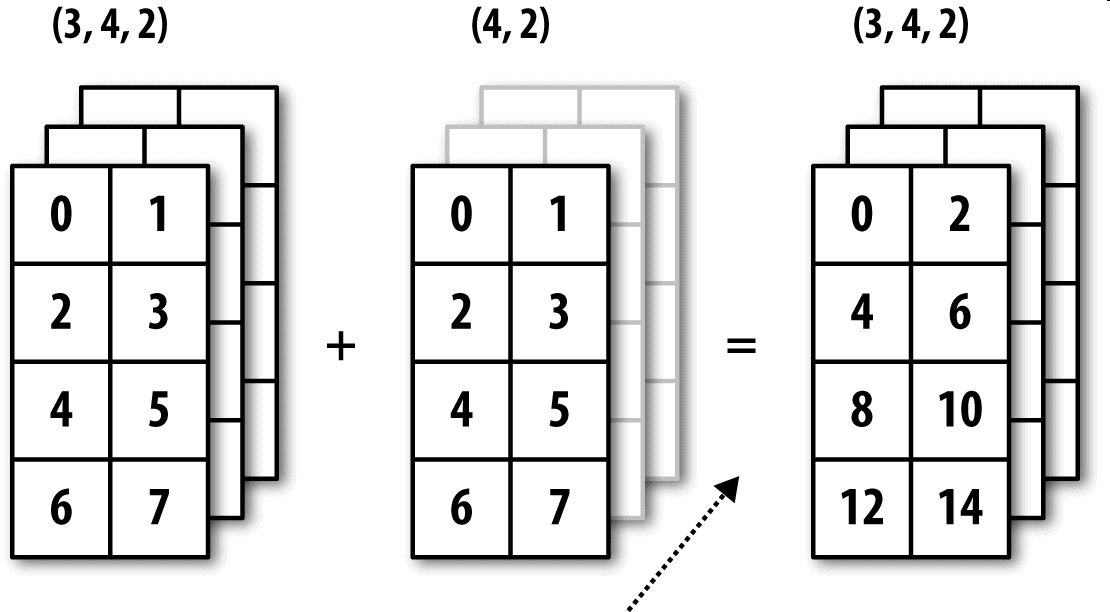沿其它轴向广播

In : arr - arr.mean(1)
---------------------------------------------------------------------------
ValueError                                Traceback (most recent call last)
<ipython-input-93-7b87b85a20b2> in <module>()
----> 1 arr - arr.mean(1)
ValueError: operands could not be broadcast together with shapes (4,3) (4,)

In : arr - arr.mean(1).reshape((4, 1))
Out:
array([[-0.2095,  1.1334, -0.9239],
[ 0.8562, -0.6828, -0.1734],
[-0.3386,  1.0823, -0.7438],
[ 0.3234, -0.8599,  0.5365]])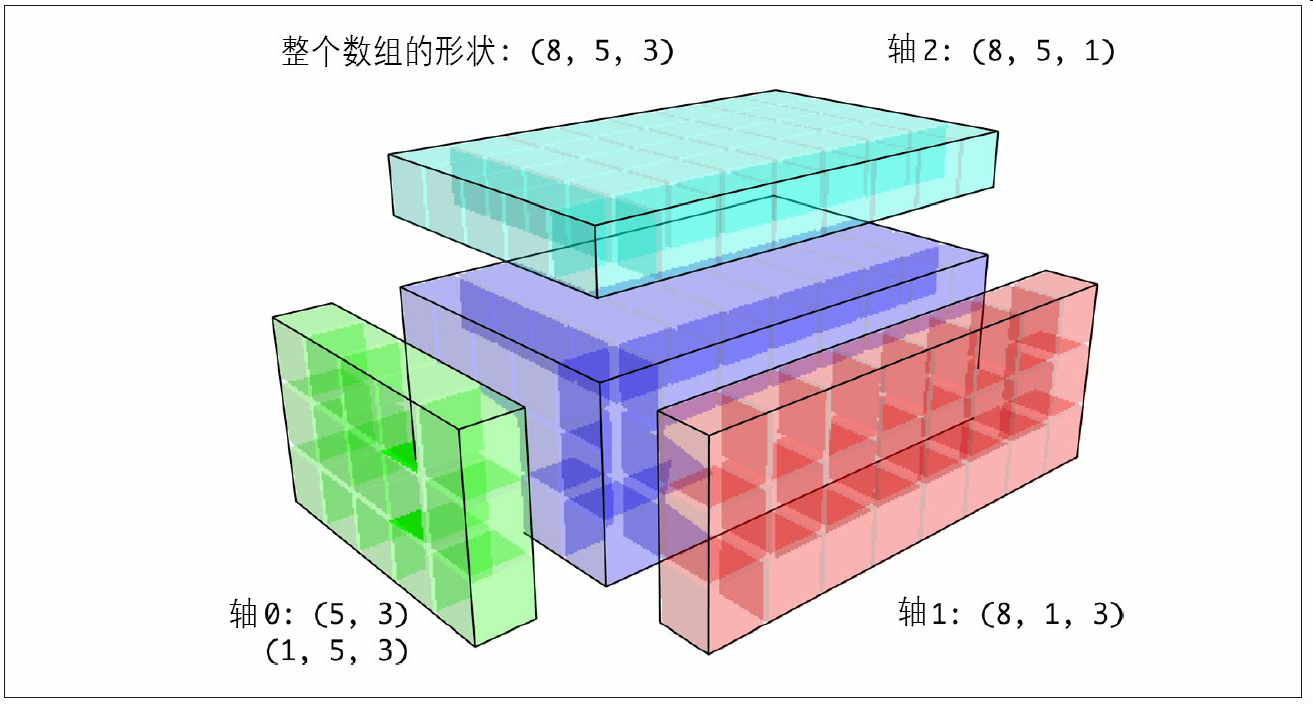In : arr = np.zeros((4, 4))

In : arr_3d = arr[:, np.newaxis, :]

In : arr_3d.shape
Out: (4, 1, 4)

In : arr_1d = np.random.normal(size=3)

In : arr_1d[:, np.newaxis]
Out:
array([[-2.3594],
[-0.1995],
[-1.542 ]])

In : arr_1d[np.newaxis, :]
Out: array([[-2.3594, -0.1995, -1.542 ]])

In : arr = np.random.randn(3, 4, 5)

In : depth_means = arr.mean(2)

In : depth_means
Out:
array([[-0.4735,  0.3971, -0.0228,  0.2001],
[-0.3521, -0.281 , -0.071 , -0.1586],
[ 0.6245,  0.6047,  0.4396, -0.2846]])

In : depth_means.shape
Out: (3, 4)

In : demeaned = arr - depth_means[:, :, np.newaxis]

In : demeaned.mean(2)
Out:
array([[ 0.,  0., -0., -0.],
[ 0.,  0., -0.,  0.],
[ 0.,  0., -0., -0.]])

def demean_axis(arr, axis=0):
means = arr.mean(axis)

# This generalizes things like [:, :, np.newaxis] to N dimensions
indexer = [slice(None)] * arr.ndim
indexer[axis] = np.newaxis
return arr - means[indexer]

通过广播设置数组的值

In : arr = np.zeros((4, 3))

In : arr[:] = 5

In : arr
Out:
array([[ 5.,  5.,  5.],
[ 5.,  5.,  5.],
[ 5.,  5.,  5.],
[ 5.,  5.,  5.]])

In : col = np.array([1.28, -0.42, 0.44, 1.6])
In : arr[:] = col[:, np.newaxis]

In : arr
Out:
array([[ 1.28,  1.28,  1.28],
[-0.42, -0.42, -0.42],
[ 0.44,  0.44,  0.44],
[ 1.6 ,  1.6 ,  1.6 ]])

In : arr[:2] = [[-1.37], [0.509]]

In : arr
Out:
array([[-1.37 , -1.37 , -1.37 ],
[ 0.509,  0.509,  0.509],
[ 0.44 ,  0.44 ,  0.44 ],
[ 1.6  ,  1.6  ,  1.6  ]])

A.4 ufunc高级应用

ufunc实例方法

NumPy的各个二元ufunc都有一些用于执行特定矢量化运算的特殊方法。表A-2汇总了这些方法，下面我将通过几个具体的例子对它们进行说明。

In : arr = np.arange(10)

Out: 45

In : arr.sum()
Out: 45

In : np.random.seed(12346)  # for reproducibility

In : arr = np.random.randn(5, 5)

In : arr[::2].sort(1) # sort a few rows

In : arr[:, :-1] < arr[:, 1:]
Out:
array([[ True,  True,  True,  True],
[False,  True, False, False],
[ True,  True,  True,  True],
[ True, False,  True,  True],
[ True,  True,  True,  True]], dtype=bool)

In : np.logical_and.reduce(arr[:, :-1] < arr[:, 1:], axis=1)
Out: array([ True, False,  True, False,  True], dtype=bool)

ccumulate跟reduce的关系就像cumsum跟sum的关系那样。它产生一个跟原数组大小相同的中间“累计”值数组：

In : arr = np.arange(15).reshape((3, 5))

Out:
array([[ 0,  1,  3,  6, 10],
[ 5, 11, 18, 26, 35],
[10, 21, 33, 46, 60]])

outer用于计算两个数组的叉积：

In : arr = np.arange(3).repeat([1, 2, 2])

In : arr
Out: array([0, 1, 1, 2, 2])

In : np.multiply.outer(arr, np.arange(5))
Out:
array([[0, 0, 0, 0, 0],
[0, 1, 2, 3, 4],
[0, 1, 2, 3, 4],
[0, 2, 4, 6, 8],
[0, 2, 4, 6, 8]])

outer输出结果的维度是两个输入数据的维度之和：

In : x, y = np.random.randn(3, 4), np.random.randn(5)

In : result = np.subtract.outer(x, y)

In : result.shape
Out: (3, 4, 5)

In : arr = np.arange(10)

In : np.add.reduceat(arr, [0, 5, 8])
Out: array([10, 18, 17])

In : arr = np.multiply.outer(np.arange(4), np.arange(5))

In : arr
Out:
array([[ 0,  0,  0,  0,  0],
[ 0,  1,  2,  3,  4],
[ 0,  2,  4,  6,  8],
[ 0,  3,  6,  9, 12]])

In : np.add.reduceat(arr, [0, 2, 4], axis=1)
Out:
array([[ 0,  0,  0],
[ 1,  5,  4],
[ 2, 10,  8],
[ 3, 15, 12]])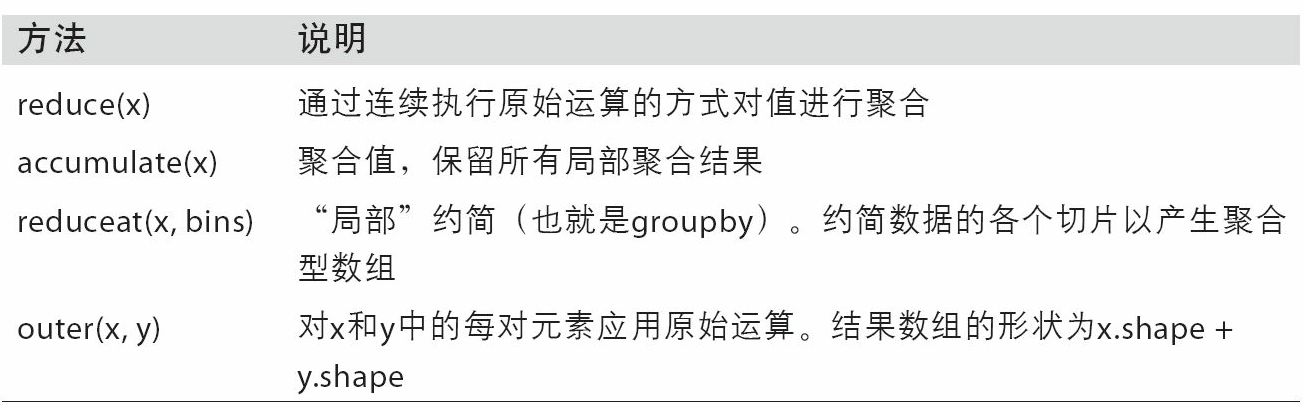编写新的ufunc

numpy.frompyfunc接受一个Python函数以及两个分别表示输入输出参数数量的参数。例如，下面是一个能够实现元素级加法的简单函数：

.....:     return x + y

Out: array([0, 2, 4, 6, 8, 10, 12, 14], dtype=object)

Out: array([  0.,   2.,   4.,   6.,   8.,  10.,  12.,  14.])

In : arr = np.random.randn(10000)

4.12 ms +- 182 us per loop (mean +- std. dev. of 7 runs, 100 loops each)

6.89 us +- 504 ns per loop (mean +- std. dev. of 7 runs, 100000 loops each)

A.5 结构化和记录式数组

In : dtype = [('x', np.float64), ('y', np.int32)]

In : sarr = np.array([(1.5, 6), (np.pi, -2)], dtype=dtype)

In : sarr
Out:
array([( 1.5   ,  6), ( 3.1416, -2)],
dtype=[('x', '<f8'), ('y', '<i4')])

In : sarr
Out: ( 1.5, 6)

In : sarr['y']
Out: 6

In : sarr['x']
Out: array([ 1.5   ,  3.1416])

嵌套dtype和多维字段

In : dtype = [('x', np.int64, 3), ('y', np.int32)]

In : arr = np.zeros(4, dtype=dtype)

In : arr
Out:
array([([0, 0, 0], 0), ([0, 0, 0], 0), ([0, 0, 0], 0), ([0, 0, 0], 0)],
dtype=[('x', '<i8', (3,)), ('y', '<i4')])

In : arr['x']
Out: array([0, 0, 0])

In : arr['x']
Out:
array([[0, 0, 0],
[0, 0, 0],
[0, 0, 0],
[0, 0, 0]])

In : dtype = [('x', [('a', 'f8'), ('b', 'f4')]), ('y', np.int32)]

In : data = np.array([((1, 2), 5), ((3, 4), 6)], dtype=dtype)

In : data['x']
Out:
array([( 1.,  2.), ( 3.,  4.)],
dtype=[('a', '<f8'), ('b', '<f4')])

In : data['y']
Out: array([5, 6], dtype=int32)

In : data['x']['a']
Out: array([ 1.,  3.])

pandas的DataFrame并不直接支持该功能，但它的分层索引机制跟这个差不多。

A.6 更多有关排序的话题

In : arr = np.random.randn(6)

In : arr.sort()

In : arr
Out: array([-1.082 ,  0.3759,  0.8014,  1.1397,  1.2888,  1.8413])

In : arr = np.random.randn(3, 5)

In : arr
Out:
array([[-0.3318, -1.4711,  0.8705, -0.0847, -1.1329],
[-1.0111, -0.3436,  2.1714,  0.1234, -0.0189],
[ 0.1773,  0.7424,  0.8548,  1.038 , -0.329 ]])

In : arr[:, 0].sort()  # Sort first column values in-place

In : arr
Out:
array([[-1.0111, -1.4711,  0.8705, -0.0847, -1.1329],
[-0.3318, -0.3436,  2.1714,  0.1234, -0.0189],
[ 0.1773,  0.7424,  0.8548,  1.038 , -0.329 ]])

In : arr = np.random.randn(5)

In : arr
Out: array([-1.1181, -0.2415, -2.0051,  0.7379, -1.0614])

In : np.sort(arr)
Out: array([-2.0051, -1.1181, -1.0614, -0.2415,  0.7379])

In : arr
Out: array([-1.1181, -0.2415, -2.0051,  0.7379, -1.0614])

In : arr = np.random.randn(3, 5)

In : arr
Out:
array([[ 0.5955, -0.2682,  1.3389, -0.1872,  0.9111],
[-0.3215,  1.0054, -0.5168,  1.1925, -0.1989],
[ 0.3969, -1.7638,  0.6071, -0.2222, -0.2171]])

In : arr.sort(axis=1)

In : arr
Out:
array([[-0.2682, -0.1872,  0.5955,  0.9111,  1.3389],
[-0.5168, -0.3215, -0.1989,  1.0054,  1.1925],
[-1.7638, -0.2222, -0.2171,  0.3969,  0.6071]])

In : arr[:, ::-1]
Out:
array([[ 1.3389,  0.9111,  0.5955, -0.1872, -0.2682],
[ 1.1925,  1.0054, -0.1989, -0.3215, -0.5168],
[ 0.6071,  0.3969, -0.2171, -0.2222, -1.7638]])

间接排序：argsort和lexsort

In : values = np.array([5, 0, 1, 3, 2])

In : indexer = values.argsort()

In : indexer
Out: array([1, 2, 4, 3, 0])

In : values[indexer]
Out: array([0, 1, 2, 3, 5])

In : arr = np.random.randn(3, 5)

In : arr = values

In : arr
Out:
array([[ 5.    ,  0.    ,  1.    ,  3.    ,  2.    ],
[-0.3636, -0.1378,  2.1777, -0.4728,  0.8356],
[-0.2089,  0.2316,  0.728 , -1.3918,  1.9956]])

In : arr[:, arr.argsort()]
Out:
array([[ 0.    ,  1.    ,  2.    ,  3.    ,  5.    ],
[-0.1378,  2.1777,  0.8356, -0.4728, -0.3636],
[ 0.2316,  0.728 ,  1.9956, -1.3918, -0.2089]])

lexsort跟argsort差不多，只不过它可以一次性对多个键数组执行间接排序（字典序）。假设我们想对一些以姓和名标识的数据进行排序：

In : first_name = np.array(['Bob', 'Jane', 'Steve', 'Bill', 'Barbara'])

In : last_name = np.array(['Jones', 'Arnold', 'Arnold', 'Jones', 'Walters'])

In : sorter = np.lexsort((first_name, last_name))

In : sorter
Out: array([1, 2, 3, 0, 4])

In : zip(last_name[sorter], first_name[sorter])
Out: <zip at 0x7fa203eda1c8>

其他排序算法

In : values = np.array(['2:first', '2:second', '1:first', '1:second',
.....:                    '1:third'])

In : key = np.array([2, 2, 1, 1, 1])

In : indexer = key.argsort(kind='mergesort')

In : indexer
Out: array([2, 3, 4, 0, 1])

In : values.take(indexer)
Out:
array(['1:first', '1:second', '1:third', '2:first', '2:second'],
dtype='<U8')

mergesort（合并排序）是唯一的稳定排序，它保证有O(n log n)的性能（空间复杂度），但是其平均性能比默认的quicksort（快速排序）要差。表A-3列出了可用的排序算法及其相关的性能指标。大部分用户完全不需要知道这些东西，但了解一下总是好的。部分排序数组

In : np.random.seed(12345)

In : arr = np.random.randn(20)

In : arr
Out:
array([-0.2047,  0.4789, -0.5194, -0.5557,  1.9658,  1.3934,  0.0929,
0.2817,  0.769 ,  1.2464,  1.0072, -1.2962,  0.275 ,  0.2289,
1.3529,  0.8864, -2.0016, -0.3718,  1.669 , -0.4386])

In : np.partition(arr, 3)
Out:
array([-2.0016, -1.2962, -0.5557, -0.5194, -0.3718, -0.4386, -0.2047,
0.2817,  0.769 ,  0.4789,  1.0072,  0.0929,  0.275 ,  0.2289,
1.3529,  0.8864,  1.3934,  1.9658,  1.669 ,  1.2464])

In : indices = np.argpartition(arr, 3)

In : indices
Out:
array([16, 11,  3,  2, 17, 19,  0,  7,  8,  1, 10,  6, 12, 13, 14, 15,  5,
4, 18,  9])

In : arr.take(indices)
Out:
array([-2.0016, -1.2962, -0.5557, -0.5194, -0.3718, -0.4386, -0.2047,
0.2817,  0.769 ,  0.4789,  1.0072,  0.0929,  0.275 ,  0.2289,
1.3529,  0.8864,  1.3934,  1.9658,  1.669 ,  1.2464])

numpy.searchsorted：在有序数组中查找元素

searchsorted是一个在有序数组上执行二分查找的数组方法，只要将值插入到它返回的那个位置就能维持数组的有序性：

In : arr = np.array([0, 1, 7, 12, 15])

In : arr.searchsorted(9)
Out: 3

In : arr.searchsorted([0, 8, 11, 16])
Out: array([0, 3, 3, 5])

In : arr = np.array([0, 0, 0, 1, 1, 1, 1])

In : arr.searchsorted([0, 1])
Out: array([0, 3])

In : arr.searchsorted([0, 1], side='right')
Out: array([3, 7])

In : data = np.floor(np.random.uniform(0, 10000, size=50))

In : bins = np.array([0, 100, 1000, 5000, 10000])

In : data
Out:
array([ 9940.,  6768.,  7908.,  1709.,   268.,  8003., 9037.,   246.,
4917.,  5262.,  5963.,   519.,  8950.,  7282.,  8183.,  5002.,
8101.,   959.,  2189.,  2587.,  4681.,  4593.,  7095.,  1780.,
5314.,  1677.,  7688.,  9281.,  6094.,  1501.,  4896.,  3773.,
8486.,  9110.,  3838.,  3154.,  5683.,  1878.,  1258.,  6875.,
7996.,  5735.,  9732.,  6340.,  8884.,  4954.,  3516.,  7142.,
5039.,  2256.])

In : labels = bins.searchsorted(data)

In : labels
Out:
array([4, 4, 4, 3, 2, 4, 4, 2, 3, 4, 4, 2, 4, 4, 4, 4, 4, 2, 3, 3, 3, 3, 4,
3, 4, 3, 4, 4, 4, 3, 3, 3, 4, 4, 3, 3, 4, 3, 3, 4, 4, 4, 4, 4, 4, 3,
3, 4, 4, 3])

In : pd.Series(data).groupby(labels).mean()
Out:
2     498.000000
3    3064.277778
4    7389.035714
dtype: float64

A.7 用Numba编写快速NumPy函数

Numba是一个开源项目，它可以利用CPUs、GPUs或其它硬件为类似NumPy的数据创建快速函数。它使用了LLVM项目（http://llvm.org/），将Python代码转换为机器代码。

import numpy as np

def mean_distance(x, y):
nx = len(x)
result = 0.0
count = 0
for i in range(nx):
result += x[i] - y[i]
count += 1
return result / count

In : x = np.random.randn(10000000)

In : y = np.random.randn(10000000)

In : %timeit mean_distance(x, y)
1 loop, best of 3: 2 s per loop

In : %timeit (x - y).mean()
100 loops, best of 3: 14.7 ms per loop

NumPy的版本要比它快过100倍。我们可以转换这个函数为编译的Numba函数，使用numba.jit函数：

In : import numba as nb

In : numba_mean_distance = nb.jit(mean_distance)

@nb.jit
def mean_distance(x, y):
nx = len(x)
result = 0.0
count = 0
for i in range(nx):
result += x[i] - y[i]
count += 1
return result / count

In : %timeit numba_mean_distance(x, y)
100 loops, best of 3: 10.3 ms per loop

Numba不能编译Python代码，但它支持纯Python写的一个部分，可以编写数值算法。

Numba是一个深厚的库，支持多种硬件、编译模式和用户插件。它还可以编译NumPy Python API的一部分，而不用for循环。Numba也可以识别可以便以为机器编码的结构体，但是若调用CPython API，它就不知道如何编译。Numba的jit函数有一个选项，nopython=True，它限制了可以被转换为Python代码的代码，这些代码可以编译为LLVM，但没有任何Python C API调用。jit(nopython=True)有一个简短的别名numba.njit。

from numba import float64, njit

@njit(float64(float64[:], float64[:]))
def mean_distance(x, y):
return (x - y).mean()

用Numba创建自定义numpy.ufunc对象

from numba import vectorize

@vectorize
return x + y

In : x = np.arange(10)

Out: array([  0.,   2.,   4.,   6.,   8.,  10.,  12.,  14.,  16.,  18.])

Out: array([  0.,   1.,   3.,   6.,  10.,  15.,  21.,  28.,  36.,  45.])

A.8 高级数组输入输出

内存映像文件

In : mmap = np.memmap('mymmap', dtype='float64', mode='w+',
.....:                  shape=(10000, 10000))

In : mmap
Out:
memmap([[ 0.,  0.,  0., ...,  0.,  0.,  0.],
[ 0.,  0.,  0., ...,  0.,  0.,  0.],
[ 0.,  0.,  0., ...,  0.,  0.,  0.],
...,
[ 0.,  0.,  0., ...,  0.,  0.,  0.],
[ 0.,  0.,  0., ...,  0.,  0.,  0.],
[ 0.,  0.,  0., ...,  0.,  0.,  0.]])

In : section = mmap[:5]

In : section[:] = np.random.randn(5, 10000)

In : mmap.flush()

In : mmap
Out:
memmap([[ 0.7584, -0.6605,  0.8626, ...,  0.6046, -0.6212,  2.0542],
[-1.2113, -1.0375,  0.7093, ..., -1.4117, -0.1719, -0.8957],
[-0.1419, -0.3375,  0.4329, ...,  1.2914, -0.752 , -0.44  ],
...,
[ 0.    ,  0.    ,  0.    , ...,  0.    ,  0.    ,  0.    ],
[ 0.    ,  0.    ,  0.    , ...,  0.    ,  0.    ,  0.    ],
[ 0.    ,  0.    ,  0.    , ...,  0.    ,  0.    ,  0.    ]])

In : del mmap

In : mmap = np.memmap('mymmap', dtype='float64', shape=(10000, 10000))

In : mmap
Out:
memmap([[ 0.7584, -0.6605,  0.8626, ...,  0.6046, -0.6212,  2.0542],
[-1.2113, -1.0375,  0.7093, ..., -1.4117, -0.1719, -0.8957],
[-0.1419, -0.3375,  0.4329, ...,  1.2914, -0.752 , -0.44  ],
...,
[ 0.    ,  0.    ,  0.    , ...,  0.    ,  0.    ,  0.    ],
[ 0.    ,  0.    ,  0.    , ...,  0.    ,  0.    ,  0.    ],
[ 0.    ,  0.    ,  0.    , ...,  0.    ,  0.    ,  0.    ]])

HDF5及其他数组存储方式

PyTables和h5py这两个Python项目可以将NumPy的数组数据存储为高效且可压缩的HDF5格式（HDF意思是“层次化数据格式”）。你可以安全地将好几百GB甚至TB的数据存储为HDF5格式。要学习Python使用HDF5，请参考pandas线上文档。

A.9 性能建议

• 将Python循环和条件逻辑转换为数组运算和布尔数组运算。
• 尽量使用广播。
• 避免复制数据，尽量使用数组视图（即切片）。
• 利用ufunc及其各种方法。

连续内存的重要性

In : arr_c = np.ones((1000, 1000), order='C')

In : arr_f = np.ones((1000, 1000), order='F')

In : arr_c.flags

Out:
C_CONTIGUOUS : True
F_CONTIGUOUS : False
OWNDATA : True
WRITEABLE : True
ALIGNED : True
UPDATEIFCOPY : False

In : arr_f.flags
Out:
C_CONTIGUOUS : False
F_CONTIGUOUS : True
OWNDATA : True
WRITEABLE : True
ALIGNED : True
UPDATEIFCOPY : False

In : arr_f.flags.f_contiguous
Out: True

In : %timeit arr_c.sum(1)
784 us +- 10.4 us per loop (mean +- std. dev. of 7 runs, 1000 loops each)

In : %timeit arr_f.sum(1)
934 us +- 29 us per loop (mean +- std. dev. of 7 runs, 1000 loops each)

In : arr_f.copy('C').flags
Out:
C_CONTIGUOUS : True
F_CONTIGUOUS : False
OWNDATA : True
WRITEABLE : True
ALIGNED : True
UPDATEIFCOPY : False

In : arr_c[:50].flags.contiguous
Out: True

In : arr_c[:, :50].flags
Out:
C_CONTIGUOUS : False
F_CONTIGUOUS : False
OWNDATA : False
WRITEABLE : True
ALIGNED : True
UPDATEIFCOPY : FalsePython3获取股票行情数据（中国个股/中国指数/全球指数）
#!/usr/local/bin/python3 #coding=utf-8 #source http://www.cnblogs.com/txw1958/ import os, io, sys, re, time, json, base64 import webbrowser, urllib.
1349 01693 0python 代码统计文件的行数
669 0python/pandas/numpy（十二）数据加载、存储与文件格式

1059 0Cray将集成分析套件带入高端超级计算机
872 0【Python数据挖掘课程】六.Numpy、Pandas和Matplotlib包基础知识

6642 01355 0CDN高级技术专家周哲：深度剖析短视频分发过程中的用户体验优化技术点

5109 0《Python数据挖掘：概念、方法与实践》——1.2节如何进行数据挖掘
1320 0718 0
seancheney

117

0

《2021云上架构与运维峰会演讲合集》

《零基础CSS入门教程》

《零基础HTML入门教程》